• 最小二乘拟合程序matlab源代码，matlab线性最小二乘逼近的求法求解！！
• 线性最小二乘数据拟合(高斯—牛顿法)当变量之间为非线性相关时，可用非线性最小二乘数据拟合(高斯—牛顿法)。[beta,r,j]=nlinfit(x,y, ′fun′,beta0)[ypred,delta]=nlpredci(FUN,inputs,beta,r,j)ci=nlparci(beta...
非线性最小二乘数据拟合(高斯—牛顿法)当变量之间为非线性相关时，可用非线性最小二乘数据拟合(高斯—牛顿法)。[beta,r,j]=nlinfit(x,y, ′fun′,beta0)[ypred,delta]=nlpredci(FUN,inputs,beta,r,j)ci=nlparci(beta,r,j)nlintool(x,y, ′fun′,beta0)nlinfit  非线性拟合函数。beta是以x,y为数据返回的系数值。fun是系数向量和数组x的函数，返回拟合y值的向量。beta0为选取的初始值向量，r为拟合残差，j为Jacobian矩阵值。nlpredci  非线性最小二乘预测置信区间。nlparci  非线性模型参数置信区间。ypred为预测值，delta为置信区间的半长值，inputs为矩阵。ci为b的误差估计。nlintool  非线性拟合交互式图形工具。显示95%置信区间上下的两条红线和其间的拟合曲线。移动纵向虚线可显示不同的自变量及其对应的预测值。还可有其他参数。;例题1:;用MATLAB编程;输入;结果;例2  已知浮煤累计灰分及产率为x和y，试进行非线性拟合。;输入    x=x’；b0=[0.2  0  -0.4  -0.1  5]；%初始值[b,r,j]=nlinfit(x,y, ′mf′,b0)；[yp,d]=nlpredci (′mf′,x,b,r,j);nlintool(x,y, ′mf′,b0);结果;第三讲 线性规划;补充知识 :解方程或方程组; 求解x2+xy+y=3，x为参数。solve(′x ^ 2+x * y+y=3′, ′x′)ans=[-1/2 * y+1/2 * (y ^ 2-4 * y+12) ^ (1/2)][-1/2 * y-1/2 * (y ^ 2-4 * y+12) ^ (1/2)];解au2+υ2=0, u-υ=1。[u,v]=solve (′a * u ^ 2+v ^ 2=0′, ′u-v=1′)u=  [1/2/(a+1) * (-2 * a+2 * (-a) ^ (1/2))+1]  [1/2/(a+1) * (-2 * a-2 * (-a) ^ (1/2))+1]v=[1/2/(a+1) 8 (-2 * a +2 * (-a) ^ (1/2))][1/2/(a+1) 8 (-2 * a -2 * (-a) ^ (1/2))];2 解线性方程组;例题;a=[1  2  1；2  2  3；-1  -3  0]；% rank(a)=3，有惟一解b=[0  3  2]’;x=linsolve(a,b)x=1-11;3 非线性方程;非线性方程解例题;非线性方程组求解;非线性方程组求解例题;程序及做法:;x=1.0000  -1.0000  1.0000fval=1.0e-006 * -0.2729  0.0810  -0.1294exitflag=1output=firstorderopt:2.9957e-008iterations:4func-count:17cgiterations:3algorithm:[1x  43  char];求解下列非线性方程组x(1)-0.7 * sin(x(1))-0.2 * cos(x(2))=0x(2)-0.7 * sin(x(1))+0.2 * cos(x(2))=0函数文件 function f=xzz(x);f(1)=x(1)-0.7 * sin (x(1))-0.2 * cos(x(2));f(2)=x(2)-0.7 * sin (x(1))+0.2 * cos(x(2));f=[f(1)  f(2)];输入   x0=[1  1]；x=fsolve(′xzz′，x0)x=0.5265  0.5079;线性规划问题是目标函数和约束条件均为线性函数的问题;函数  linprog格式  x = linprog(f,A,b)   %求min  f ' *x   sub.to  线性规划的最优解。x = linprog(f,A,b,Aeq,beq)   %等式约束，若没有不等式约束，则A=[ ]，b=[ ]。x = linprog(f,A,b,Aeq,beq,lb,ub)   %指定x的范围，若没有等式约束 ，则Aeq=[ ]，beq=[ ]x = linprog(f,A,b,Aeq,beq,lb,ub,x0)   %设置初值x0x = linprog(f,A,b,Aeq,beq,lb,ub,x0,options)    % options为指定的优化参数[x,fval] = linprog(…)   % 返回目标函数最优值，即fval= f ' *x
展开全文• Matlab最小二乘拟合图像；可以拟合任意数量的数据；可查看截距、斜率；有网格；之线区间可以根据自己实际需求改写，默认600；显示线性度的最大非线性；有图例，说明哪个数据是什么
• 利用MATLAB最小二乘拟合线性函数，求取其中的几个参数，有么有大神会啊。谢谢了函数 源代码
• 用一个实例来理解基于最小二乘的非线性拟合问题。 原理部分 代码部分 clear; clc; M = 2000; t = 0.3 * (1 : M)'; rng('default'); ratio = 10; noise = ratio * randn(M, 1); Et = 1000 * exp(-t / 50) + 10 ...
用一个实例来理解基于最小二乘的非线性拟合问题。

原理部分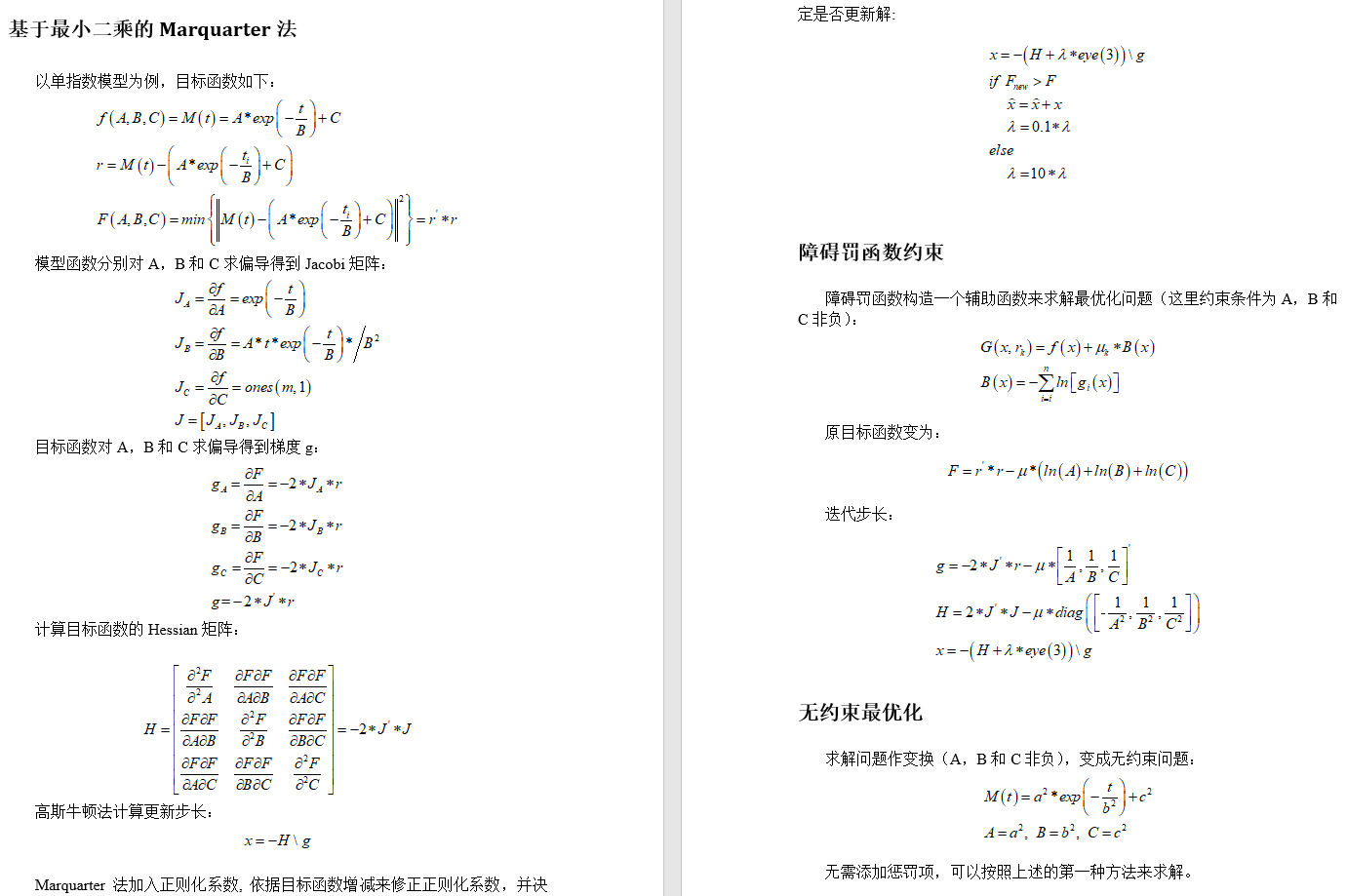代码部分

clear; clc;
M = 2000;
t = 0.3 * (1 : M)';
rng('default');
ratio = 10;
noise = ratio * randn(M, 1);
Et = 1000 * exp(-t / 50) + 10 + noise;
p1 = LSFittingT2Free(t, Et);
p2 = LSFittingT2Penalty(t, Et);
fit1 = p1(1) * exp(-t / p1(2)) + p1(3);
fit2 = p2(1) * exp(-t / p2(2)) + p2(3);
disp([p1; p2]);
plot(t, Et, '.k', 'LineWidth', 1.5)
hold on
plot(t, fit1, 'LineWidth', 1.5)
plot(t, fit2, 'LineWidth', 1.5)
hold off
legend('Model', 'Fitting1', 'Fitting2')

function result = LSFittingT2Free(x, y)
% Levenberg_Marquardt LS Fitting
% y = a^2 * exp(-x / b^2) + c^2
%{
clear; clc;
M = 2000;
t = 0.3 * (1 : M)';
rng('default');
ratio = 10;
noise = ratio * randn(M, 1);
Et = 1000 * exp(-t / 50) + 10 + noise;
p = LSFittingT2Free(t, Et);
fit = p(1) * exp(-t / p(2)) + p(3);
plot(t, Et, '.k', 'LineWidth', 1.5)
hold on
plot(t, fit, 'LineWidth', 1.5)
hold off
legend('Model', 'Fitting')
%}
% 按列排
x = x(:);
y = y(:);
% 归一化
maxValue = max(y);
y = y / maxValue;
M = length(x);
% Least Squares Minimization
rng('default');
% 初始值
a = sqrt(rand);
b = sqrt(rand);
c = sqrt(rand);
% 变量个数
nParam = 3;
% 残差
r = y - (a^2 * exp(-x / b^2) + c^2);
% 目标函数
f = r'*r;
% 正则化因子初值
lambda = 1;
% 迭代次数
it = 0;
% 更新标记
updateFlag = true;
% 最大迭代次数
maxIter = 100;
% 迭代计算
while it < maxIter
it = it + 1;
if updateFlag
Ja = 2 * a * exp(-x / b^2);
Jb = 2 * a^2 * x .* exp(-x / b^2) / b^3;
Jc = 2 * c * ones(M, 1);
J = [Ja, Jb, Jc];
g = -2 * J' * r;
H = 2 * (J'*J);
end
Hess = H + lambda * eye(nParam);
s = -Hess \ g;
a1 = a + s(1);
b1 = b + s(2);
c1 = c + s(3);
r1 = y - (a1^2 * exp(-x / b1^2) + c1^2);
f1 = r1'*r1;
fdr = (f - f1) / f;
if fdr > 0
a = a1;
b = b1;
c = c1;
f = f1;
r = r1;
lambda = 0.1 * lambda;
updateFlag = true;
else
lambda = 10 * lambda;
updateFlag = false;
end
if max(abs(s)) < 1e-6
disp(['反演收敛，迭代次数：' num2str(it)]);
break;
elseif it == maxIter
disp('反演可能不收敛；');
end
end
result = [a^2 * maxValue, b^2, c^2 * maxValue];

function result = LSFittingT2Penalty(x, y)
% Levenberg_Marquardt LS Fitting
% y = a^2 * exp(-x / b^2) + c^2
%{
clear; clc;
M = 2000;
t = 0.3 * (1 : M)';
rng('default');
ratio = 10;
noise = ratio * randn(M, 1);
Et = 1000 * exp(-t / 50) + 10 + noise;
p = LSFittingT2Free(t, Et);
fit = p(1) * exp(-t / p(2)) + p(3);
plot(t, Et, '.k', 'LineWidth', 1.5)
hold on
plot(t, fit, 'LineWidth', 1.5)
hold off
legend('Model', 'Fitting')
%}
% 按列排
x = x(:);
y = y(:);
M = length(x);
% 初始值
a0 = rand;
b0 = rand;
c0 = rand;
% 变量个数
nParam = 3;
% 残差
r0 = y - (a0 * exp(-x / b0) + c0);
% 障碍罚函数的收缩系数个数
numLoop = 5;
% 最大迭代次数
maxIter = 100;
% 障碍罚函数收缩系数
mu = 10;
k = 0;
while k < numLoop
k = k + 1;
mu = mu * 0.1;
% Least Squares Minimization
a = a0;
b = b0;
c = c0;
r = r0;
% 目标函数值
f = r'*r - mu * (log(a) + log(b) + log(c));
% 正则化因子初值
lambda = 1;
% 迭代次数
it = 0;
% 更新标记
updateFlag = true;
% 迭代计算
while it < maxIter
it = it + 1;
if updateFlag
Ja = exp(-x / b);
Jb = a * x .* exp(-x / b) / b^2;
J = [Ja, Jb, ones(M, 1)];
g = -2 * J' * r - mu * [1/a; 1/b; 1/c];
H = 2 * (J'*J) - mu * diag([-1/a^2, -1/b^2, -1/c^2]);
end
s = -(H + lambda * eye(nParam)) \ g;
while a + s(1) < 0 || b + s(2) < 0 || c + s(3) < 0
s = 0.5 * s;
end
a1 = a + s(1);
b1 = b + s(2);
c1 = c + s(3);
r1 = y - (a1 * exp(-x / b1) + c1);
f1 = (r1'*r1) - mu * (log(a1) + log(b1) + log(c1));
fdr = (f - f1) / f;
if fdr > 0
a = a1;
b = b1;
c = c1;
f = f1;
r = r1;
lambda = 0.1 * lambda;
updateFlag = true;
else
lambda = 10 * lambda;
updateFlag = false;
end
if max(abs(s)) < 1e-6
disp(['反演收敛，迭代次数：' num2str(it)]);
break;
elseif it == maxIter
disp('反演可能不收敛；');
end
end
history.a(k) = a;
history.b(k) = b;
history.c(k) = c;
history.ssr(k) = r'*r;
end
[~, minIndex] = min(history.ssr);
a = history.a(minIndex);
b = history.b(minIndex);
c = history.c(minIndex);
result = [a, b, c];


结果部分

程序输出结果如下，和Matlab拟合工具箱结果对比，完全一致。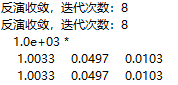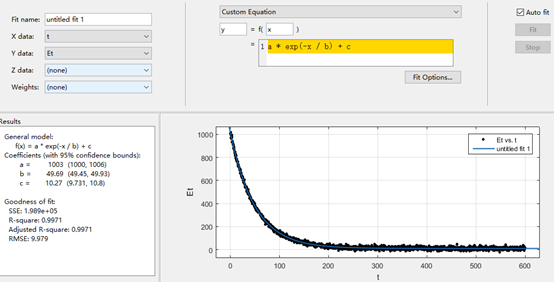展开全文• 突然有个想法，利用机器学习的基本方法——线性回归方法，来学习一阶rc电路的阶跃响应，从而得到rc电路的结构特征——时间常数τ(即r*c)。回答无疑是肯定的，但问题是怎样通过最小二乘法、正规方程，以更多的采样...
突然有个想法，利用机器学习的基本方法——线性回归方法，来学习一阶rc电路的阶跃响应，从而得到rc电路的结构特征——时间常数τ(即r*c)。回答无疑是肯定的，但问题是怎样通过最小二乘法、正规方程，以更多的采样点数来降低信号采集噪声对τ估计值的影响。另外，由于最近在捣鼓jupyter和numpy这些东西，正好尝试不用matlab而用jupyter试试看。结果是意外的好用，尤其是在jupyter脚本中插入latex格式的公式的功能，真是太方便了！尝试了直接把纸上手写的公式转换到jupyter脚本中的常见工具软件。以下原创内容欢迎网友转载，但请注明出处：一、理论推导1．线性回归分析及正规方程传统意义说，线性回归问题是用最小二乘法(即正规方程)，解决线性方程组的均方误差最小化问题。已知输出输入x是由多个变量构成的行向量，w是系数向量(列向量)，b为偏置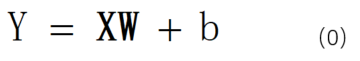在机器学习中，把每次的输入x作为一行组成更大的矩阵，即每一行代表一个样本，该矩阵称为设计矩阵x(train)。若样本数为k，则x(train)有k行，每个样本都会得到一个输出y，将y集合成一个列向量y(train)，k个相同的b也组成列向量b。为简化表达，将b简化为附加在系数列向量w最后的常数b，x(train)则在每行的最后增加一个1，w(列向量)的最后增加一个待估计的b。为了使估计的结果：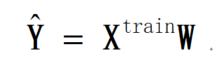和y(train)之差的平方和最小，有正规方程可以求解w: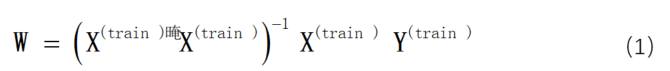2.一阶rc电路的阶跃响应一阶rc电路的电路模型如下图所示。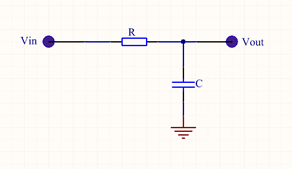图1 一阶rc电路幅度为vcc的阶跃信号从vin处输入，在vout处测量输出。解微分方程可得自变量为时间t的响应函数。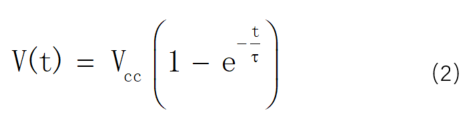其中时间常数τ = r*c。我希望通过测量阶跃信号输入条件下，实际rc电路的响应曲线v(t)，并通过v(t)来估计时间常数τ。如果做纯理论推导，只要知道任意时刻t0的输出电压v(t0)就可以解方程(2)得到τ。但在实际电路中电压v(t0)的测量往往受到诸多干扰的影响，并不准确。是否可以测量多个t值时刻对应的v(t)，并利用正规方程(1)得到一个统计意义上最优的估计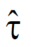呢？是接下来要解决的问题。3.非线性函数的最小二乘估计仔细观察适用正规方程的目标函数(0)式的特点，可以发想让非线性的要让(2)式能够使用正规方程，必须让：1)     含有待估计的变量τ的函数充当(0)式中的系数w，而设计矩阵x(train)则可以由含有时间t或测量电压v(t)的函数充当。2)     w和x(train)之间的关系必须是简单的相乘。显然，只有用时间t的序列充当设计矩阵x(train)，才有可能使w和x(train)之间的关系必须是相乘。至于其他的非线性部分则可以通过等效变换，变换到等式的另一侧来充当y(train)。综上，可以将(2)式变换为(3)式。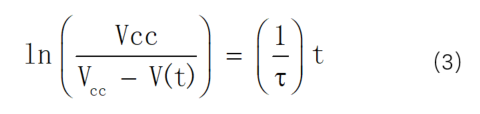(3)式的整个左边可以计算得到y(train)；(3)式右边的时间t的序列在构成设计矩阵x(train)，1/τ则构成相当于(0)式中的系数矩阵w。这样就可以通过正规方程(2)式来求解统计意义上最优的估计了。当然，从(3)式也可以看出，经过线性校正的模型中系数向量w只有一个元素——是个标量，偏置b也是恒等于0的。二、仿真模型想利用最近正在尝试使用的jupyter和numpy替代熟悉的matlab，验证上述非线性函数最小二乘估计的想法。下面先建立一个模型：1)     输入为幅度vcc为3.3v的阶跃信号；2)     时间常数τ为0.1秒；3)     简单模拟采样间隔的随机性：是间隔等于delta1=0.0015秒和delta1=0.0011秒的两个序列的叠加。4)     采样总长度为n=5倍τ；5)     信号上叠加了幅度约为20mv的白噪声——至于为什么是20mv，将在后续部分详细介绍。利用python和numpy进行数值仿真的代码如下：1 import numpy as np2 import matplotlib.pyplot as plt3 tao=0.1#时间常数4 vcc=3.3#电源电压5 n=5#时长取时间常数tao的n倍6 delta1=0.0015#第一种采样间隔7 delta2=0.0011#第一种采样间隔8 t1=delta1*np.arange(np.ceil(n*tao/delta1))9 t2=delta2*np.arange(np.ceil(n*tao/delta2))10 t=np.append(t1,t2)#为演示最小二乘拟合的功能，故意结合两种采样率下的时间点11 t.sort()#对t进行排序12 plt.plot(t)13 s=vcc*(1-np.exp(-t/tao))#理论的波形曲线14 plt.plot(t,s)#注意这里的plot函数使用了x轴和y轴两个轴，因为s中的数据不是均匀的15 n_amp=np.exp(-n)*vcc16 n_amp17 noise = np.random.uniform(-n_amp, n_amp, (len(t)))#噪声：正负np.exp(-5)*3.3之间均匀分布18 s_nr=s+noise#加入噪声后的信号19 plt.plot(t,s_nr)20 yt=np.log(vcc/(vcc-s_nr))21 plt.plot(t,yt)22 yt=np.mat(yt)23 yt=yt.t24 x=np.mat(t)#将x转换为矩阵数据类型25 x=x.t#正规方程中的x应该是个列向量26 w=(np.linalg.inv(x.t*x))*x.t*yt#求解正规方程27 e_tao = np.round(1/float(w),4)#对时间常数的tao的估计，保留到4位小数28 e_tao说明：1)     其间序列包含了两个等差数列t1和t2的融合，它们的间隔互质，没有重复，目的是模拟采样时间的随机性。最后用sort()方法又对时间序列进行排序的目的是为了后续容易观察波形更直观。如果仅仅为了使用正规方程，是不需要重新排序的。2)     校正的非线性方程(3)和原始方程(2)相比有一个重大的缺陷就是：左侧求对数的括号内的数值不能为负——如果是纯理论推导，这是不可能发生的。但假如随机噪声后的v(t)是有可能大于阶跃幅度vcc的，此时括号内将变为一个负数，使得计算变得没有意义。我的解决之道是将假如的随机噪声幅度限制在仿真截止时刻v(t)和vcc之差的范围内，及代码中的n_amp。由于仿真的结束时刻为n(=5)个τ，所以n_amp的值等于np.exp(-n)*vcc。这样做没有任何理论依据，仅仅是受限于(3)和(2)式之间的非完全等价变换——属于线性化校正需要付出的代价。3)     由于待估计的参数只有一个(1/τ)所以正规方程的解也是只有一个元素的矩阵。将其转换为标量后取倒得到最优估计。三、结果评估加入噪声后的信号如下图所示，与通常情况的实测波形吻合度很高。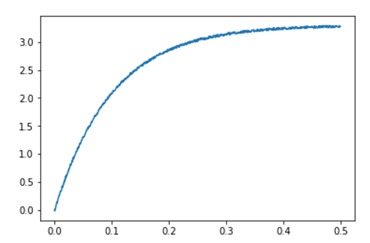图2 模拟产生的带有噪声的阶跃响应对这些加入噪声的信号进行线性校正后得到进行最小二乘估计的信号yt为下图所示。其基本趋势是一条斜率为(1/τ)的直线，和我预计的结果一样。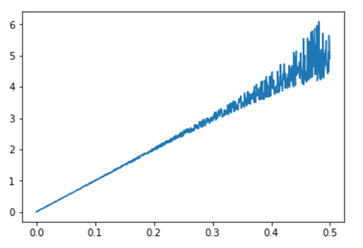图3 对图2进行线性校正后的待估计信号但可以明显的看到，由于(3)式左侧在v(t)的大小接近vcc时对加入的白噪声进行了放大。因此当t递增时，由白噪声造成的信号的不确定性大大增加了。也就是在套用正规方程时，t值较大时的噪声对结果的影响大于t值较小时的噪声对结果的影响。这是使用非线性校正函数需要付出的重要代价。另外，多次运行以上代码的得到 都是一个略小于实际τ(=0.1)的数值——约为0.099左右，也就是说， 不是无偏估计。这应该是由于线性校正函数((3)式左侧)，对于噪声noise大于0和小于0的部分进行了非对称的变换造成的。这虽然造成的偏差不大，但也是使用非线性校正函数需要付出的代价。四、jupyter notebook上述练习的一个重要目的是练习使用jupyter notebook，并在其中内嵌具有很好交互性的公式等信息。以下是部分程序运行效果的截图，虽然我对markdown语法还不熟悉，格式和排版还不够漂亮，但还是能够明显的提高代码的可读性。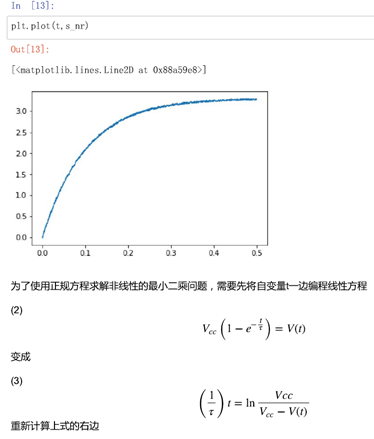图4 jupyter notebook运行效果截图其中需要重点记录下的是公式代码的嵌入过程：1)我首先在纸上手写了一些公式，用手机对其拍照，如：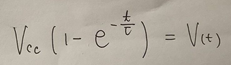图5 手写的公式2)用mathpix tools对这些照片截图，并扫描(mathpix tools有windows版和ios版，均可免费试用)。mathpix可以直接得到latex格式的公式表达式。下图是ios版本的mathpix界面截图。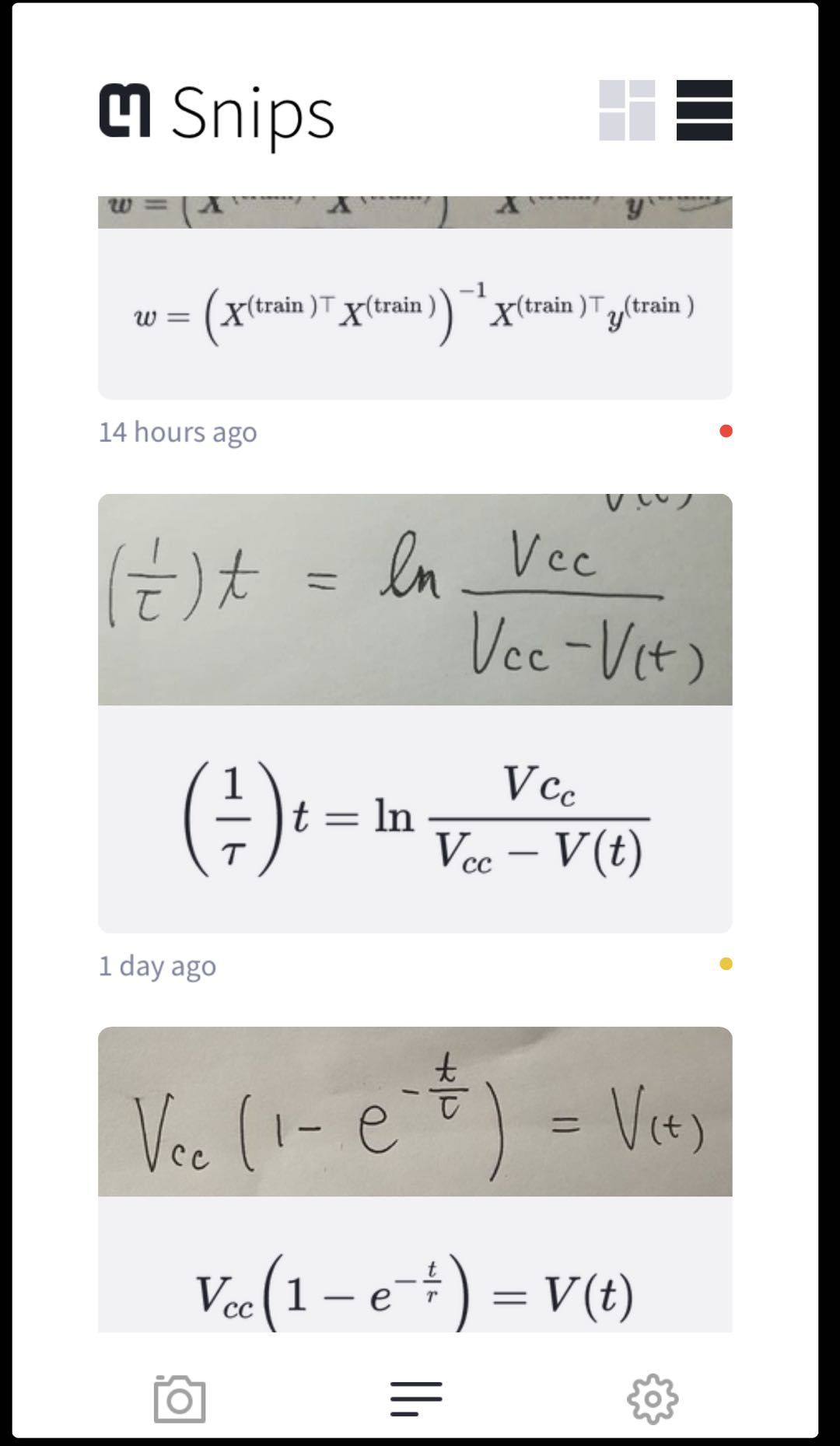图6 ios版本的mathpix截图3)在jupyter notebook上部的下拉菜单中选择单元格的格式为markdown；将latex格式的公式表达式粘贴到该单元格内，并在latex公式表达式的前后加上“”符号，运行该单元格即可得到图4所示效果的公式了。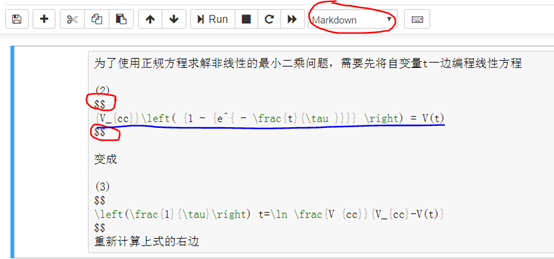图7 在jupyter notebook中输入latex公式五、进一步的实际测试工作做到这里离其实并没有完，还应该做一个简单的实际电路实测一下。我会在后续的假期中抽半天时间，在stm32开发板上完成这个工作：用gpio产生一个节约信号后，连续采集5个τ时间长度的信号，并代入正规方程求解，欢迎大家继续关注更新。……希望与广大网友互动？？点此进行留言吧！
展开全文• 1、线性最小二乘拟合 最小二乘法（又称最小平方法）是一种数学优化技术，其通过最小化误差的平方和寻找数据的最佳函数匹配。利用最小二乘法可以简便地求得未知的数据，并使得这些求得的数据与实际数据之间误差的...
1、线性最小二乘拟合
最小二乘法（又称最小平方法）是一种数学优化技术，其通过最小化误差的平方和寻找数据的最佳函数匹配。利用最小二乘法可以简便地求得未知的数据，并使得这些求得的数据与实际数据之间误差的平方和为最小。最小二乘法通过变量的数据来描述变量之间的相互关系。例如通过描述x、y之间的相互关系。
常见的多项式拟合曲线有：直线、多项式、双曲线、指数曲线。Matlab中的最小二乘函数：P=polyfit(x,y,n)（n=1时为），[P Smu]=polyfit(x,y,n)，polyval(P,t)返回n次多项式在t处的值（plot(t,
polyval(P,t)）。P-返回n次拟合多项式系数从高到低依次存放于向量P中，S-包含三个值其中normr是残差平方和，mu-包含两个值mean（x）均值，std（x）标准差。
2、非线性拟合
超定方程组（方程组的个数大于未知数的个数），Matlab中提供lsqcurvefit和lsqnonlin两个非线性最小二乘拟合函数，两者的区别在于其定于的函数f(x)不一样。
非线性曲线拟合lsqcurvefit用以求含参量x（向量）的向量值函数F(x,xdata)=（F（x，xdata1），…，F（x，xdatan））T中的参变量x(向量)，使得：
最小
其输入格式有：
(1)x = lsqcurvefit (‘fun’,x0,xdata,ydata);
(2)x =lsqcurvefit (‘fun’,x0,xdata,ydata,options);
(4)[x, options] = lsqcurvefit (‘fun’,x0,xdata,ydata,…);
(5)[x, options,funval] = lsqcurvefit (‘fun’,x0,xdata,ydata,…);
(6)[x, options,funval, Jacob] = lsqcurvefit (‘fun’,x0,xdata,ydata,…);
其中fun为事先建立的函数F(x,xdata)的M-文件x0为迭代初始值，xdata、ydata为已知数据点、options为无约束优化。
非线性最小二乘拟合lsqnonlin用以求含参量x（向量）的向量值函数f(x)=(f1(x),f2(x),…,fn(x))T中的参量x，使得：
最小
其中
其输入格式为：
(1)x=lsqnonlin(‘fun’,x0);
(2)x= lsqnonlin (‘fun’,x0,options);
(4)[x，options]=lsqnonlin(‘fun’,x0,dwx,upx,options,Y);
其中，fun为建立的M文件，x0为初始值，dwx、upx为上、下限，options为无约束优化，Y为传入fun的参数值。

无约束优化options的设定options=optimset(‘optionName’,’optionValue’)，例如options = optimset('Display','iter','TolFun',1e-8)使得优化函数值的误差为1e-8，iter改为off不显示迭代过程。options = optimset('param1',value1,'param2',value2,...)

展开全文迭代 优化
• 最小二乘法拟合方法一般线性最小二乘拟合方法非线性最小二乘拟合方法 基本定义的叙述这里笔者给出网址，读者可自行点击前往阅读。 最小二乘法...算法
• 线性拟合 下面举一个拟合年份和人口的例子 年份从1790-2000以十年的间隔递增，具体代码见下： x=[3.9 5.3 7.2 9.6 12.9 17.1 23.2 31.4 38.6 50.2 62.9 76 92 106.5 123.2 131.7 150.7 179.3 204 226.5 251.4 281....
• 上传资源包含椭圆拟合matlab程序及其用到的英文文档理论，用最小二乘线性迭代的方法拟合椭圆。函数返回的数据较多，如重心坐标，长轴，短轴，倾斜角度和椭圆拟合函数。
• 摘 要文章简单介绍了Matlab与Mathematica的拟合函数功能，并通过具体例子的数据，分别利用Matlab与Mathematica进行了非线性拟合，对比了它们的应用办法，分析了各自的特点。关键词数据拟合；Matlab；Mathematica；内...
• 最近由于工作需要要做一套线性方程的拟合算法，在网上找了很多，不是用gsl就是用matlab做的，都不符合要求。实在找不到合适的于是就决定自己从头写，于是自己写了一个，用了一段时间决得还行，就决定贴出来和大家...源代码 百度云 算法
• 设x,y,z是空间点的坐标，R为一系列坐标点上对应的...我现在想用最小二乘法非线性拟合，实现当我给定一个R时，找到对应的（x,y,z）. 我不知道三维的又没有函数表达式该怎么求，用lsqnonlin能实现吗？请各位大神指教。开发语言
• 解决非线性最小二乘(非线性数据拟合)问题 解决非线性最小二乘曲线拟合问题的形式 变量x的约束上下限为ub和lb， x = lsqnonlin(fun,x0)从x0点开始，找到fun中描述的函数的最小平方和。函数fun应该返回一个向量(或...
• 月只蓝以我的了解，Levenberg...直接调用该函数，用默认的算法即可，如果出现算法设置不合理，MATLAB会提示你的，到时候你换另外一个就行了。飞鸿印雪jay引用回帖:月只蓝 at 2014-08-25 21:15:24以我的了解，Levenbe...
• 原文：matlab中的偏最小二乘回归（PLSR）和主成分回归（PCR）​tecdat.cn此示例显示如何在matlab中应用偏最小二乘回归（PLSR）和主成分回归（PCR），并讨论这两种方法的有效性。当存在大量预测变量时，PLSR和PCR都是...
• 突然有个想法，利用机器学习的基本方法——线性回归方法，来学习一阶RC电路的阶跃响应，从而得到RC电路的结构特征——时间常数τ（即R*C）。回答无疑是肯定的，但问题是怎样通过最小二乘法、正规方程，以更多的采样...
• 1;2;3;4;5;6;7;8;9;10;11;12;13;14;15;16;17;18;19;20;21;22;23;24;25;26;27;28;29;给药方案 ;...用线性最小二乘拟合c(t;给药方案 设计;34;35;36;37;38;39;40;41;42;43;44;45;46;47;48;49;50;51;52;53
• 可以方便地进行矩阵的分解，求解线性方程组，以及进行多项式操作等。在输入矩阵时可 以直接输入数学表达式包括复数，例如可以直接输入sin(i+1)^2，而不同提前计算具体数值 。可以直接输入pi。使用说明在压缩包里。...
• min  s.t. v1xv2 求解程序名为lsqnonlin，其最简单的调用格式为： x=lsqnonlin(@F,x0, v1,v2) 其最复杂的调用格式为： ...[x,norm,res,ef,out,lam,...l 非线性拟合问题 min  s.t. v1xv2 求解程序名为lsqcurve...
• 线性拟合matlab仿真代码，包含数据点的收集、一般最小二乘算法、正交回归算法，画图等。其中数据点的收集还包括曲线的数据点收集。
• min s.t. v1xv2 求解程序名为lsqnonlin，其最简单的调用格式为： x=lsqnonlin(@F,x0, v1,v2) 其最复杂的调用格式为： ...l 非线性拟合问题 min s.t. v1xv2 求解程序名为lsqcurvefit，其最简单的调用格式...
• 1.线性最小二乘法曲线拟合问题的提法是，已知一组(二维)数据，即平面上的个点...拟合准则是使，与的距离 的平方和最小，称为最小二乘准则。1.1.系数的确定记：为求使达到最小，只需利用极值的必要条件，得到关于的线...
• 最小二乘法主要用于解决函数模型最优解问题，是测量工作及其他科学工程领域中，应用最早也是最广泛的算法。 在生产实践中，经常会遇到利用一组观测数据来估计某些未知参数的问题。 举个栗子， 一个做匀速运动的...
• ## matlab线性拟合

千次阅读 2014-06-03 17:14:17
原文地址：matlab线性拟合作者：Txping7 11.1 曲线拟合 曲线拟合涉及回答两个基本问题：最佳拟合意味着什么？应该用什么样的曲线？可用许多不同的方法定义最佳...数学上，称为多项式的最小二乘曲线拟合。如果这种
• 通过Matlab实现偏最小二乘法，最小二乘法可以在非线性拟合中进行应用
• 最小二乘是建立表到表的线性拟合关系，然后预测的方法(处理高维数据)，比如在光谱分析中，X是某物质的光谱样本构成的训练集，Y是对应的成分数据，x是要预测成分的光谱数据。
• 根据解的存在情况，线性方程可以分为:有唯一解的恰定方程组，解不存在的超定方程组，有无穷多...对于超定方程，在MATLAB中，利用左除命令(x=A\b)来寻求它的最小二乘解；还可以用广义逆来求，即x=pinv(A),所得的解 不...
• ## matlab lsqcurvefit 非线性拟合

万次阅读 多人点赞 2018-08-19 20:28:35
线性曲线拟合是已知输入...今进行曲线拟合，求x使得输出的如下最小二乘表达式成立： 例 1 拟合如下非线性函数 函数曲线如下所示。 fit_fun.m function diff = fit_fun(param, X) a = param(1); m = pa...
• 1、最小二乘原理 　Matlab直接实现最小二乘法的示例：　 close x = 1:1:100; a = -1.5; b = -10; y = a*log(x)+b;...%%最小二乘拟合 xf=log(x); yf=yrand; xfa = [ones(1,size(xf,2));xf]......

# 最小二乘线性拟合matlabmatlab 订阅